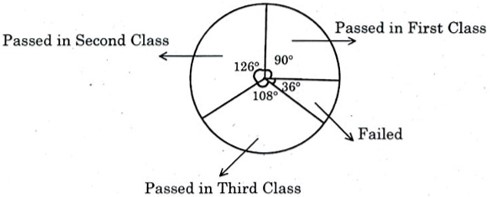# Percentage TNPSC Group 4 VAO Questions

## Percentage MCQ Questions

1.

Raman buys a washing machine for Rs.13,500 and sells it at a loss of 12%.What is the selling price of the washing machine?

VAO - 2016

A.

11,880

B.

11,800

C.

13,500

D.

11,870

A. 11,880

2.

A bicycle marked at Rs. 1,500 is sold for Rs. 1,350. What is the percentage of discount?

Group 1 - 2015

A.

12

B.

15

C.

11

D.

10

D. 10

3.

Due to increase of 30% in the price of a color TV the sale is reduced by 40%. What will be the percentage change in income?

Group 1 - 2019

A.

10% increase

B.

10% decrease

C.

35% decrease

D.

22% decrease

D. 22% decrease

4.Given the pie chart. Obtain the pass percentage of student who passed in 1st class

Group 2 - 2018

A.

35%

B.

30%

C.

10%

D.

25%

D. 25%

5.

A man sells two wrist watches at Rs. 594 each. On one he gains 10% and on the other he loses 10%. Find his gain or loss percent on the whole

Group 2 - 2018

A.

Loss % = 90%

B.

Gain % = 5%

C.

Loss % = 1%

D.

Loss % = 7%

C. Loss % = 1%

6.

The price of a house is decreased from rupees fifteen lakhs to rupees twelve lakhs. The percentage of decrease is

Group 4 - 2016

A.

10%

B.

20%

C.

30%

D.

40%